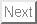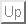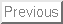Next: Introduction

# Approximation of distribution function of a generalized likelihood ratio for the detection of spatial partially coherent signals in the short sample case

O. V. Bolkhovskaya*, A. A. Maltsev*, L. Lo Presti**, F. Sellone**

email

*Department of Statistical Radiophisics, Nizhny Novgorod State University

**Dipartimento di Elettronica, Politecnico di Torino

Received 06 December 2003

### Abstract:

The generalized likelihood ratio (GLR) method uses a likelihood ratio
as a test-statistic, in which all unknown parameters of a signal and noise are substituted by them ML-estimates. In general, obtained test-statistichas a complicate probability distribution. This does not allow to find analytically the test-statistic threshold
for the given constant level of false alarm probability
. Therefore, various numerical or asymptotic methods can be adopted. Unfortunately such methods perform well only when the number of collected samples is large, hereafter referred as large sample case. In the present work the constant-false-alarm-rate (CFAR) detection task of multidimensional Gaussian complex signals with unknown spatial covariance matrix on a background of additive Gaussian complex noise of a unknown power is solved for the case where only few samples are collected, hereafter referred as small sample case. In spite of the fact that the distribution function of the random variableis not represented in an analytical form, the exact analytical expressions for statistical moments of any order for the function
were found. On the next step the approximating series for probability density function (PDF)of the random variable
was constructed with the help of the beta probability distribution and orthogonal Jacobi polynomials on the base of known test-statistichigher orders moments. The accuracy of the test-statisticcumulative distribution function (CDF) approximation and the accuracy of threshold
calculations on the given
for various numbers of approximating series terms were checked by simulation. It is shown, that the employed approximating series has very high rate of convergence.Next: Introduction